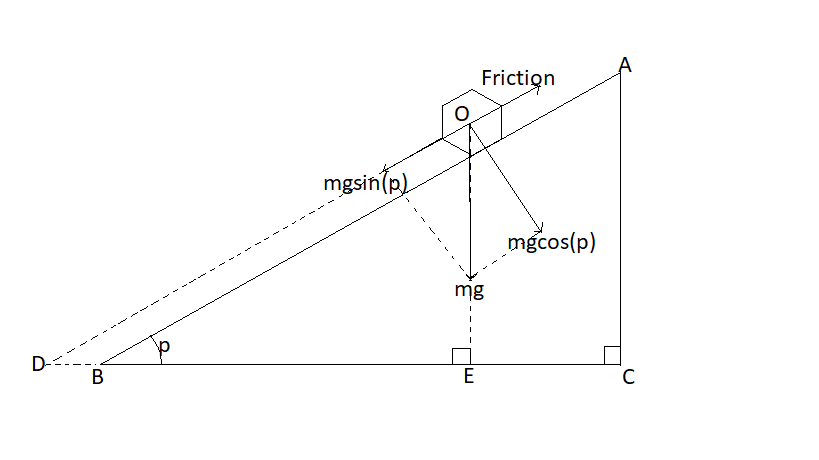# It's slope!

After working for sometime on friction I got the following result:

Suppose there is a box on top of a tilted surface and coefficient of static friction between them is $µ$. Now suppose the angle at which the surface is inclined to ground is $\theta$ and you are increasing it slowly, after reaching a certain value $\theta=p$ the force of friction reaches it's maximum point (i.e. if you now increase $\theta$ a little more, the box will fall). Then the following relation holds true: $µ=\tan(p)$

Proof:

Let the mass of the box be $m$

The diagram below will be very helpful:The force of friction acting $=µN=µmg\cos(p)$

As net force is $0$, therefore $\cancel{mg}\sin(p)=µ\cancel{mg}\cos(p)$ $\sin(p)=µ\cos(p)$ $\boxed{µ=\tan(p)}$

Note:

• I haven't proved the diagram because I found it very easier to proof, if you want you can proof it yourself and write it in the comment it can be challenge for you!Note by Zakir Husain
1 year ago

This discussion board is a place to discuss our Daily Challenges and the math and science related to those challenges. Explanations are more than just a solution — they should explain the steps and thinking strategies that you used to obtain the solution. Comments should further the discussion of math and science.

When posting on Brilliant:

• Use the emojis to react to an explanation, whether you're congratulating a job well done , or just really confused .
• Ask specific questions about the challenge or the steps in somebody's explanation. Well-posed questions can add a lot to the discussion, but posting "I don't understand!" doesn't help anyone.
• Try to contribute something new to the discussion, whether it is an extension, generalization or other idea related to the challenge.
• Stay on topic — we're all here to learn more about math and science, not to hear about your favorite get-rich-quick scheme or current world events.

MarkdownAppears as
*italics* or _italics_ italics
**bold** or __bold__ bold
- bulleted- list
• bulleted
• list
1. numbered2. list
1. numbered
2. list
Note: you must add a full line of space before and after lists for them to show up correctly
paragraph 1paragraph 2

paragraph 1

paragraph 2

[example link](https://brilliant.org)example link
> This is a quote
This is a quote
    # I indented these lines
# 4 spaces, and now they show
# up as a code block.

print "hello world"
# I indented these lines
# 4 spaces, and now they show
# up as a code block.

print "hello world"
MathAppears as
Remember to wrap math in $$ ... $$ or $ ... $ to ensure proper formatting.
2 \times 3 $2 \times 3$
2^{34} $2^{34}$
a_{i-1} $a_{i-1}$
\frac{2}{3} $\frac{2}{3}$
\sqrt{2} $\sqrt{2}$
\sum_{i=1}^3 $\sum_{i=1}^3$
\sin \theta $\sin \theta$
\boxed{123} $\boxed{123}$

## Comments

There are no comments in this discussion.

×

Problem Loading...

Note Loading...

Set Loading...